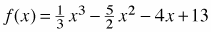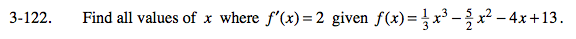### Home > CALC > Chapter 3 > Lesson 3.3.3 > Problem3-122

3-122.

Find all values of x where f ′(x) = 2 given. Homework Help ✎Since f(x) is cubic, f '(x) will be quadratic. Predict how many solutions there will be to f '(x) = 2.

The blue curve shows f '(x) intersecting the dotted line y = 2. Do your solutions look correct?

Use the Power rule to find f '(x).

Set f '(x) = 2, and solve for x.

Use the eTool below to explore the graph of f(x).
Click the link at right for the full version of the eTool: Calc 3-122 HW eTool